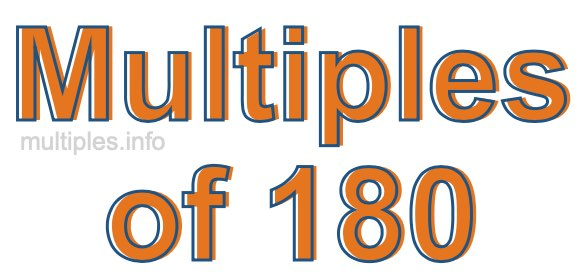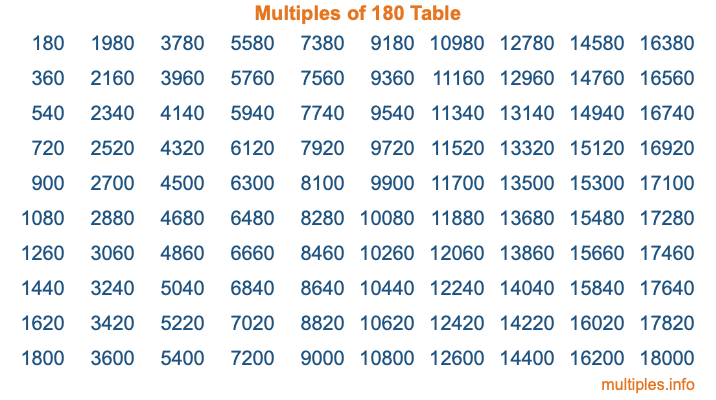Multiples of 180Welcome to the Multiples of 180 page. Here we will first teach you everything you will ever need to know about the multiples of 180, and then give you a study guide summary of everything we taught you to make sure you remember it all. Use this page to look up facts and learn information about the multiples of 180. This page will make you a multiples of one hundred eighty expert!

Definition of Multiples of 180
Multiples of 180 are all the numbers that when divided by 180 equal an integer. Each of the multiples of 180 are called a multiple. A multiple of 180 is created by multiplying 180 by an integer.

Therefore, to create a list of multiples of 180, you start with 1 multiplied by 180, then 2 multiplied by 180, then 3 multiplied by 180, and so on for as long as you want. Thus, the list of the first five multiples of 180 is 180, 360, 540, 720, and 900. To see a larger list of multiples of 180, see the printable image of Multiples of 180 further down on this page. We also have a category where you can choose any nth multiple of 180.

Multiples of 180 Checker
The Multiples of 180 Checker below checks to see if any number of your choice is a multiple of 180. In other words, it checks to see if there is any number (integer) that when multiplied by 180 will equal your number. To do that, we divide your number by 180. If the the quotient is an integer, then your number is a multiple of 180.

Is  a multiple of 180?

Least Common Multiple of 180 and ...
A Least Common Multiple (LCM) is the lowest multiple that two or more numbers have in common. This is also called the smallest common multiple or lowest common multiple and is useful to know when you are adding our subtracting fractions. Enter one or more numbers below (180 is already entered) to find the LCM.

Check out our LCM Calculator if you need more details about the Least Common Multiple or if you need the LCM for different numbers for adding and subtraction fractions.

nth Multiple of 180
As we stated above, 180 is the first multiple of 180, 360 is the second multiple of 180, 540 is the third multiple of 180, and so on. Enter a number below to find the nth multiple of 180.

th multiple of 180

Multiples of 180 vs Factors of 180
180 is a multiple of 180 and a factor of 180, but that is where the similarities end. All postive multiples of 180 are 180 or greater than 180. All positive factors of 180 are 180 or less than 180.

Below is the beginning list of multiples of 180 and the factors of 180 so you can compare:

Multiples of 180: 180, 360, 540, 720, 900, etc.

Factors of 180: 1, 2, 3, 4, 5, 6, 9, 10, 12, 15, 18, 20, 30, 36, 45, 60, 90, 180

As you can see, the multiples of 180 are all the numbers that you can divide by 180 to get a whole number. The factors of 180, on the other hand, are all the whole numbers that you can multiply by another whole number to get 180.

It's also interesting to note that if a number (x) is a factor of 180, then 180 will also be a multiple of that number (x).

Multiples of 180 vs Divisors of 180
The divisors of 180 are all the integers that 180 can be divided by evenly. Below is a list of the divisors of 180.

Divisors of 180: 1, 2, 3, 4, 5, 6, 9, 10, 12, 15, 18, 20, 30, 36, 45, 60, 90, 180

The interesting thing to note here is that if you take any multiple of 180 and divide it by a divisor of 180, you will see that the quotient is an integer.

Multiples of 180 Table
Below is an image of the first 100 multiples of 180 in a table. The table is in chronological order, column by column. The first column has the first ten multiples of 180, the second column has the next ten multiples of 180, and so on.The Multiples of 180 Table is also referred to as the 180 Times Table or Times Table of 180. You are welcome to print out our table for your studies.

Negative Multiples of 180
Although not often discussed or needed in math, it is worth mentioning that you can make a list of negative multiples of 180 by multiplying 180 by -1, then by -2, then by -3, and so on, to get the following list of negative multiples of 180:

-180, -360, -540, -720, -900, etc.

Multiples of 180 Summary
Below is a summary of important Multiples of 180 facts that we have discussed on this page. To retain the knowledge on this page, we recommend that you read through the summary and explain to yourself or a study partner why they hold true.

There are an infinite number of multiples of 180.

A multiple of 180 divided by 180 will equal a whole number.

180 divided by a factor of 180 equals a divisor of 180.

The nth multiple of 180 is n times 180.

The largest factor of 180 is equal to the first positive multiple of 180.

180 is a multiple of every factor of 180.

180 is a multiple of 180.

A multiple of 180 divided by a divisor of 180 equals an integer.

180 divided by a divisor of 180 equals a factor of 180.

Any integer times 180 will equal a multiple of 180.

Multiples of a Number
Here you can get the multiples of another number, all with the same attention to detail as we did for multiples of 180 on this page.

Multiples of
Multiples of 181
Did you find our page about multiples of one hundred eighty educational? Do you want more knowledge? Check out the multiples of the next number on our list!